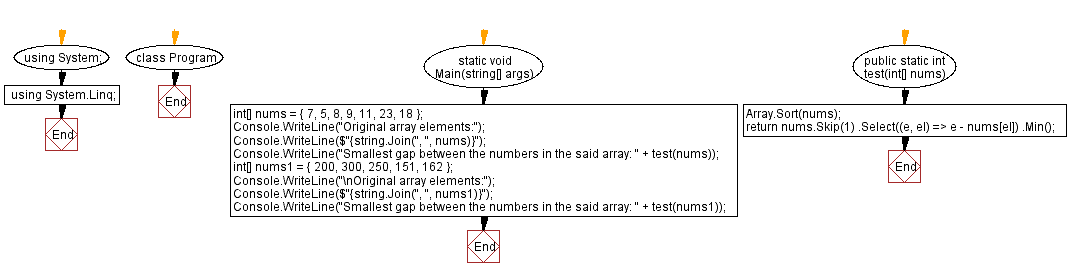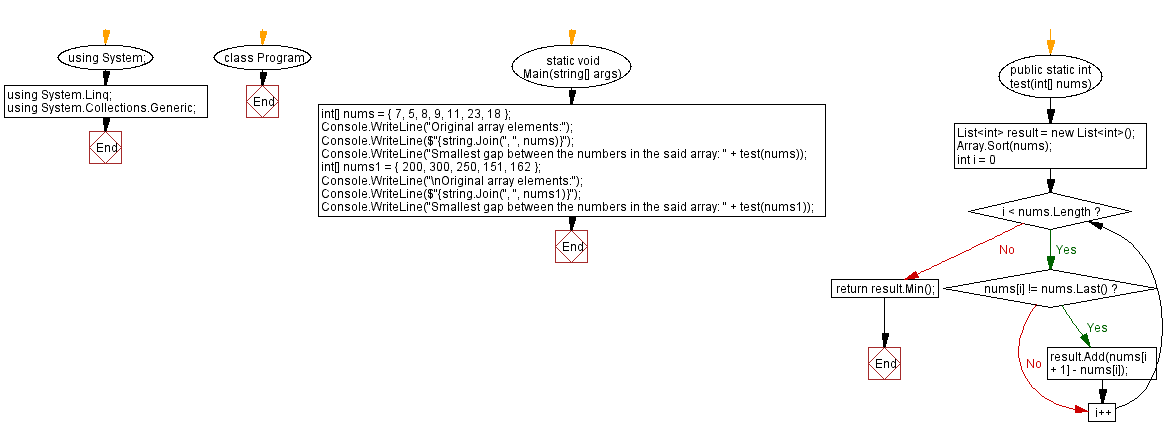﻿ C# - Smallest gap between the numbers in an array# C# Sharp Exercises: Smallest gap between the numbers in an array

## C# Sharp Array: Exercise-37 with Solution

Write a C# Sharp program that calculates the smallest gap between the numbers in an array of integers.

Sample Data:
({ 7, 5, 8, 9, 11, 23, 18 }) -> 1
({ 200, 300, 250, 151, 162 }) -> 11

Sample Solution-1:

C# Sharp Code:

``````using System;
using System.Linq;
namespace exercises
{
class Program
{
static void Main(string[] args)
{
int[] nums = { 7, 5, 8, 9, 11, 23, 18 };
Console.WriteLine("Original array elements:");
Console.WriteLine(\$"{string.Join(", ", nums)}");
Console.WriteLine("Smallest gap between the numbers in the said array: " + test(nums));
int[] nums1 = { 200, 300, 250, 151, 162 };
Console.WriteLine("\nOriginal array elements:");
Console.WriteLine(\$"{string.Join(", ", nums1)}");
Console.WriteLine("Smallest gap between the numbers in the said array: " + test(nums1));
}
public static int test(int[] nums)
{
Array.Sort(nums);
return nums.Skip(1)
.Select((e, el) => e - nums[el])
.Min();
}
}
}
```
```

Sample Output:

```Original array elements:
7, 5, 8, 9, 11, 23, 18
Smallest gap between the numbers in the said array: 1

Original array elements:
200, 300, 250, 151, 162
Smallest gap between the numbers in the said array: 11
```

Flowchart:Sample Solution-2:

C# Sharp Code:

``````using System;
using System.Linq;
using System.Collections.Generic;
namespace exercises
{
class Program
{
static void Main(string[] args)
{
int[] nums = { 7, 5, 8, 9, 11, 23, 18 };
Console.WriteLine("Original array elements:");
Console.WriteLine(\$"{string.Join(", ", nums)}");
Console.WriteLine("Smallest gap between the numbers in the said array: " + test(nums));
int[] nums1 = { 200, 300, 250, 151, 162 };
Console.WriteLine("\nOriginal array elements:");
Console.WriteLine(\$"{string.Join(", ", nums1)}");
Console.WriteLine("Smallest gap between the numbers in the said array: " + test(nums1));
}
public static int test(int[] nums)
{
List<int> result = new List<int>();
Array.Sort(nums);
for (int i = 0; i < nums.Length; i++)
if (nums[i] != nums.Last())
result.Add(nums[i + 1] - nums[i]);
return result.Min();
}
}
}
```
```

Sample Output:

```Original array elements:
7, 5, 8, 9, 11, 23, 18
Smallest gap between the numbers in the said array: 1

Original array elements:
200, 300, 250, 151, 162
Smallest gap between the numbers in the said array: 11
```

Flowchart:C# Sharp Code Editor:

Contribute your code and comments through Disqus.

Previous C# Sharp Exercise: Check the numbers of a array are consecutive or not.
Next C# Sharp Exercise: Check specific digit in an array of numbers.

What is the difficulty level of this exercise?

Test your Programming skills with w3resource's quiz.

﻿